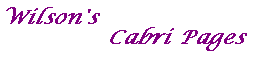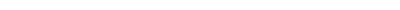Note that, if we take the points A,B',C,A',B,C' (in that order) as the vertices of a hexagon, then the line pairs (AB', A'B, etc) are pairs of opposite sides of the hexagon. Thus the theorem can be stated as If the vertices of a hexagon lie on a conic, then the intersections of opposite sides are collinear. See Brianchon's Theorem. The points do not have to be distinct. For example, if we have B'=A, then the result holds with the "chord" AB' being the tangent at A. See The 3 Tangents and 3 Chords Theorem.Tell me about Pascal Download pascal.figMain Cabri PageClassical Theorems